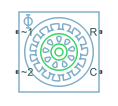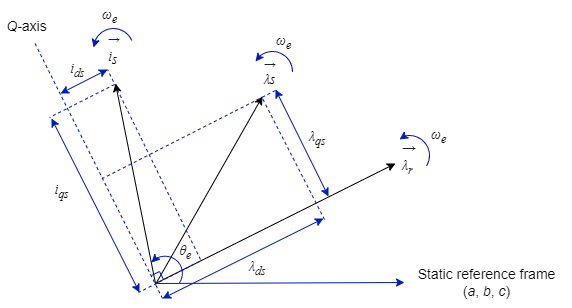# FEM-Parameterized Induction Machine (Squirrel Cage)

Squirrel-cage-rotor induction machine defined in terms of magnetic flux linkage

Since R2023a

•Libraries:
Simscape / Electrical / Electromechanical / Asynchronous

## Description

The FEM-Parameterized Induction Machine (Squirrel Cage) block models a squirrel-cage-rotor induction machine using magnetic flux linkage tables.

You can generate the magnetic flux linkage tables with a finite element tool such as ANSYS Motor-CAD software.

You can access all the stator connections on the block. Therefore, you can model soft-start regimes using a switch between wye and delta configurations.

Connect port ~1 to a three-phase circuit. To connect the stator in delta configuration, connect a Phase Permute block between ports ~1 and ~2. To connect the stator in wye configuration, connect port ~2 to a Grounded Neutral (Three-Phase) or Floating Neutral (Three-Phase) block.

### Equations

A three-phase induction machine consists of an iron stator lamination with three copper windings and a rotor with copper bars. In a squirrel-cage machine, the copper bars are shorted together.

When a three-phase AC supply feeds the stator, the current produces a rotating magnetic field. This rotating field induces currents in the rotor copper bars, which produce a magnetic field in the rotor. The interaction between these magnetic fields and the currents produces a torque in the rotor, which starts its motion.

The induction machine equations are expressed in a synchronous reference frame orientated with the rotor field (SRFORF). This frame is synchronous with the stator and rotor magnetic fields, and the D-axis is aligned with the rotor field D-axis. The rotor magnetic flux linkage vector only has a D-axis component in this frame. The Q-axis component is zero.

`${{\lambda }^{\prime }}_{qr}=0.$`• $\stackrel{\to }{{\lambda }_{s}}$ is the stator magnetic field vector.

• $\stackrel{\to }{{\lambda }_{r}}$ is the rotor magnetic field vector.

• $\stackrel{\to }{{i}_{s}}$ is the stator current vector.

All of these vectors rotate at the synchronous speed ωe. The synchronous angle θe tracks the orientation of the stator current vector.

The rotor current vector rotates at a speed given by this equation:

`${\omega }_{slip}={\omega }_{e}-{N}_{pp}{\omega }_{r},$`

where ωr is the rotor mechanical speed and Npp is the Number of pole pairs parameter.

To express the stator a-, b-, and c-phase voltages and currents in the SRFORF reference frame, you can use the Park transform:

`${P}_{s}=2/3\left[\begin{array}{ccc}\mathrm{cos}{\theta }_{e}& \mathrm{cos}\left({\theta }_{e}-2\pi /3\right)& \mathrm{cos}\left({\theta }_{e}+2\pi /3\right)\\ -\mathrm{sin}{\theta }_{e}& -\mathrm{sin}\left({\theta }_{e}-2\pi /3\right)& -\mathrm{sin}\left({\theta }_{e}+2\pi /3\right)\\ 0.5& 0.5& 0.5\end{array}\right],$`

where θe is the time integral of the synchronous speed:

`${\theta }_{e}=\int {\omega }_{e}\text{\hspace{0.17em}}dt.$`

You can then compute the D-axis and Q-axis components of stator voltages and currents using these equations.

`$\begin{array}{l}i{s}_{dq0}={P}_{s}\cdot i{s}_{abc}\\ v{s}_{dq0}={P}_{s}\cdot v{s}_{abc}\end{array}$`

The rotor a-, b-, and c-phase voltages and currents do not rotate at the synchronous speed due to slip. Rotor currents rotate at the slip speed ωslip.

To express rotor DQ currents in the stationary frame (a, b, and c phases) you can use the Park transform:

`${P}_{r}=2/3\left[\begin{array}{ccc}\mathrm{cos}{\theta }_{er}& \mathrm{cos}\left({\theta }_{er}-2\pi /3\right)& \mathrm{cos}\left({\theta }_{er}+2\pi /3\right)\\ -\mathrm{sin}{\theta }_{er}& -\mathrm{sin}\left({\theta }_{er}-2\pi /3\right)& -\mathrm{sin}\left({\theta }_{er}+2\pi /3\right)\\ 0.5& 0.5& 0.5\end{array}\right],$`

where θer is the rotor slip angle, equal to the time integral of the slip speed:

`${\theta }_{er}=\int {\omega }_{slip}\text{\hspace{0.17em}}dt.$`

Then, you can compute the a, b, and c components of stator voltages and currents using these equations.

`$\begin{array}{l}i{r}_{abc}={P}_{r}^{-1}\cdot i{r}_{dq0}\\ v{r}_{abc}={P}_{r}^{-1}\cdot v{r}_{dq0}\end{array}$`

In the SRFORF reference frame, the stator and rotor currents, voltages, and fluxes are related to these fundamental induction machine equations:

• Stator voltage equations

`$\begin{array}{l}{v}_{ds}={R}_{s}{i}_{ds}-{\omega }_{e}{\lambda }_{qs}+\frac{d{\lambda }_{ds}}{dt}\\ {v}_{qs}={R}_{s}{i}_{qs}+{\omega }_{e}{\lambda }_{ds}+\frac{d{\lambda }_{qs}}{dt}\end{array}$`

• Rotor voltage equations

`$\begin{array}{l}{{v}^{\prime }}_{dr}={{R}^{\prime }}_{r}{{i}^{\prime }}_{dr}+\frac{d{{\lambda }^{\prime }}_{dr}}{dt}\\ {{v}^{\prime }}_{qr}={{R}^{\prime }}_{r}{{i}^{\prime }}_{qr}+{\omega }_{slip}{{\lambda }^{\prime }}_{dr}\end{array}$`

Use the latter rotor voltage equation to compute the slip speed, which you can then use to compute the synchronous speed for the reference frame transformations.

`${\omega }_{slip}\text{\hspace{0.17em}}\text{\hspace{0.17em}}=\text{\hspace{0.17em}}\text{\hspace{0.17em}}\frac{{{v}^{\prime }}_{qr}-{{R}^{\prime }}_{r}{{i}^{\prime }}_{qr}}{{{\lambda }^{\prime }}_{dr}}.$`

• Squirrel cage equations: shorted rotor cage

`$\begin{array}{l}{{v}^{\prime }}_{dr}=0\\ {{v}^{\prime }}_{qr}=0\end{array}$`

• Flux-current relationships

The magnetizing D-axis current is defined as

`${i}_{d}\text{\hspace{0.17em}}=\text{\hspace{0.17em}}\text{\hspace{0.17em}}{i}_{ds}+\text{\hspace{0.17em}}\text{\hspace{0.17em}}{{i}^{\prime }}_{dr},$`

and the flux-current relationships are

`$\begin{array}{l}{{\lambda }^{\prime }}_{dr}={\lambda }_{ds}-{L}_{t}{i}_{ds}\\ {{\lambda }^{\prime }}_{qr}={\lambda }_{qs}-{L}_{t}{i}_{qs}\end{array}$`

where:

• λds is the interpolated value of the Stator d-axis flux linkage table, λds(ids,iqs) parameter computed for the particular combination of id and iqs.

• λqs is the interpolated value of the Stator q-axis flux linkage table, λqs(ids,iqs) parameter computed for the particular combination of id and iqs.

• Lt is the interpolated value of the transient inductance computed from the magnetic flux linkage tables.

An alternative form of the rotor flux-current relationships uses the Lϕ inductance

`$\begin{array}{l}{{\lambda }^{\prime }}_{dr}={L}_{\varphi }{i}_{d}\\ {{\lambda }^{\prime }}_{qr}={L}_{\varphi }{i}_{q}\end{array}$`

where Lϕ is the interpolated value of the magnetizing inductance table computed from the flux linkage tables.

When you combine the latter equation for the Q-axis rotor flux with ${{\lambda }^{\prime }}_{qr}=0$ you obtain ${i}_{q}=0$, which means that the referred rotor Q-axis current is equal and opposite to the stator Q-axis current:

`${{i}^{\prime }}_{qr}=-{i}_{qs}.$`

Lϕ is defined by this equation.

`${L}_{\varphi }={L}_{s}-{L}_{t},$`

where Lt is the transient inductance is defined as

`${L}_{t}={L}_{s}-\frac{{L}_{m}^{2}}{{{L}^{\prime }}_{r}}.$`

In these equations Ls, Lm, and ${{L}^{\prime }}_{r}$ are the stator inductance, the magnetizing inductance, and the referred rotor inductance respectively. These equations relate these inductances:

`$\begin{array}{l}{L}_{s}={L}_{ls}+{L}_{m}\\ {{L}^{\prime }}_{r}={{L}^{\prime }}_{lr}+{L}_{m}\end{array}$`

where Lls and ${{L}^{\prime }}_{lr}$ are the stator leakage inductance and the referred rotor leakage inductance, respectively.

• Torque equation

`$T=\frac{3}{2}{N}_{pp}\left({\lambda }_{ds}{i}_{qs}-{\lambda }_{qs}{i}_{ds}\right).$`

### Model Thermal Effects

You can expose thermal ports to simulate the effects of generated heat and motor temperature. To expose the thermal ports and enable the Temperature Dependence and Thermal Port parameters, set the Modeling option parameter to one of these options:

• `No thermal port` — The block contains electrical conserving ports associated with the stator windings, but does not contain thermal ports.

• `Show thermal port` — The block contains expanded electrical conserving ports associated with the stator windings and thermal conserving ports for each of the windings and for the rotor.

For more information about using thermal ports in actuator blocks, see Simulating Thermal Effects in Rotational and Translational Actuators.

### Variables

To set the priority and initial target values for the block variables before simulation, use the Initial Targets section in the block dialog box or Property Inspector. For more information, see Set Priority and Initial Target for Block Variables.

Nominal values provide a way to specify the expected magnitude of a variable in a model. Using system scaling based on nominal values increases the simulation robustness. You can specify nominal values using different sources, including the Nominal Values section in the block dialog box or Property Inspector. For more information, see System Scaling by Nominal Values.

## Ports

### Conserving

expand all

Composite three-phase port associated with the stator positive-end connections. For more information about three-phase ports, see Three-Phase Ports.

Composite three-phase port associated with the stator negative-end connections.

Mechanical rotational conserving port associated with the machine rotor.

Mechanical rotational conserving port associated with the machine case.

Thermal conserving port associated with the a-phase terminal.

#### Dependencies

To enable this port, set Modeling option to `Show thermal port`.

Thermal conserving port associated with the b-phase terminal.

#### Dependencies

To enable this port, set Modeling option to `Show thermal port`.

Thermal conserving port associated with the c-phase terminal.

#### Dependencies

To enable this port, set Modeling option to `Show thermal port`.

Thermal conserving port associated with the rotor.

#### Dependencies

To enable this port, set Modeling option to `Show thermal port`.

## Parameters

expand all

Option to enable the thermal ports of the block and model the effects of generated heat and machine temperature.

### Resistance

Stator resistance.

Option to tabulate the rotor resistance with the frequency.

Clear this parameter to specify a constant rotor resistance at the rated temperature.

Select this parameter to specify resistance as a function of frequency at the rated temperature.

Rotor resistance referred to the stator.

#### Dependencies

To enable this parameter, clear the Tabulate rotor resistance with frequency parameter.

Rotor AC frequency vector.

#### Dependencies

To enable this parameter, select the Tabulate rotor resistance with frequency parameter.

Table of rotor resistance referred to the stator as a function of the rotor AC frequency vector.

#### Dependencies

To enable this parameter, select the Tabulate rotor resistance with frequency parameter.

### Flux

Number of machine pole pairs.

Stator D-axis current vector.

Stator Q-axis current vector.

The default value corresponds to linear flux. Using linear flux is equivalent to the modeling approach of the Induction Machine Squirrel Cage block.

The default value corresponds to linear flux. Using linear flux is equivalent to the modeling approach of the Induction Machine Squirrel Cage block.

Interpolation method for approximating the output value when the input value is between grid points:

• `Linear` — Use an extension of the linear algorithm for multidimensional interpolation. Select this option to get the best performance.

• `Smooth` — Use a modified Akima interpolation algorithm. Select this option to produce a continuous surface with continuous first-order derivatives.

For more information about interpolation methods, see `tablelookup`.

### Mechanical

Option to include rotor inertia.

Rotor inertia.

#### Dependencies

To enable this parameter, select the Include rotor inertia parameter.

Rotor damping.

### Temperature Dependence

To enable these parameters, set Modeling option to `Show thermal port`.

Temperature at which you quote the motor parameters.

Coefficient α in the equation relating resistance to temperature for all three stator windings. For more information, see Thermal Model for Actuator Blocks. The default value is for copper.

Coefficient α in the equation relating resistance to temperature for the rotor. For more information, see Thermal Model for Actuator Blocks. The default value is for copper.

### Thermal Port

To enable these parameters, set Modeling option to `Show thermal port`.

Option to include stator and rotor thermal masses.

Thermal mass value for each stator winding. The thermal mass is the energy required to raise the temperature by one degree.

#### Dependencies

To enable this parameter, select the Include stator and rotor thermal masses parameter.

Thermal mass of the rotor. The thermal mass is the energy required to raise the temperature of the rotor by one degree.

#### Dependencies

To enable this parameter, select the Include stator and rotor thermal masses parameter.

 Carbonieri, Matteo, Nicola Bianchi, and Luigi Alberti. “Induction Motor Mapping Using Rotor Field-Oriented Analysis Technique.” In 2019 IEEE Energy Conversion Congress and Exposition (ECCE), 2321–28. Baltimore, MD, USA: IEEE, 2019. https://doi.org/10.1109/ECCE.2019.8912787.

 Carbonieri, Matteo, Lino Di Leonardo, Marco Tursini, Marco Villani, and Mircea Popescu. “Induction Motor Performance Prediction Using Static FEA: Method Description and Comparison With Time-Domain Approach.” In 2020 IEEE Energy Conversion Congress and Exposition (ECCE), 1351–56. Detroit, MI, USA: IEEE, 2020. https://doi.org/10.1109/ECCE44975.2020.9236246.

 Troncon, Diego, Matteo Carbonieri, Luigi Alberti, and Nicola Bianchi. “Measures and Simulations of Induction Machines Flux Linkage Characteristics Based on Rotor Field Orientation.” IEEE Transactions on Industry Applications 57, no. 5 (September 2021): 4686–93. https://doi.org/10.1109/TIA.2021.3089662.